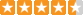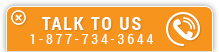# Vector Fundamentals

Course Number: S-2016
Credit: 2 PDH
Subject Matter Expert: Mark A. Strain, P.E.
Price: \$59.90 Purchase using Reward Tokens. Details34 reviews
Overview

#### In Vector Fundamentals, you'll learn ...

• What are vectors and how they are used as a mathematical tool in various engineering disciplines
• Components of a vector
• Various vector operations, including dot product and cross product

#### OverviewPreview a portion of this course before purchasing it.

Credit: 2 PDH

Length: 14 pages

Vector analysis is a mathematical tool used to explain and predict physical phenomena in the study of mechanics. A vector is a depiction or symbol showing movement or a force carried from point A to point B. A vector has properties of both magnitude and direction. A scalar only has the property of magnitude. A scalar is a quantity, like mass (14 kg), temperature (25?C), or electric field intensity (40 N/C) that only has magnitude and no direction. On the other hand, a vector has both magnitude and direction. Physical quantities that have magnitude and direction can be represented by the length and direction of an arrow.

Mechanics is the science of motion and the study of the action of forces on bodies. Mechanics is a physical science incorporating mathematical concepts directly applicable to many fields of engineering such as mechanical, civil, structural and electrical engineering. Vectors are tools used in the study of mechanics.

The purpose of this course is to describe vectors and to explain their use and to demonstrate their many applications. This course also describes several vector operations including the dot product and cross product.

#### Specific Knowledge or Skill Obtained

This course teaches the following specific knowledge and skills:

• How to apply the use of vectors to different fields of engineering
• How to decompose a vector into its individual components
• How to find the length of a vector
• How to compute the dot product of a vector
• How to compute the cross product of a vector
• How to determine the angle between two vectors

#### Certificate of Completion

You will be able to immediately print a certificate of completion after passing a multiple-choice quiz consisting of 15 questions. PDH credits are not awarded until the course is completed and quiz is passed.

Board Acceptance
 This course is applicable to professional engineers in: Alabama (P.E.) Alaska (P.E.) Arkansas (P.E.) Delaware (P.E.) Florida (P.E. Area of Practice) Georgia (P.E.) Idaho (P.E.) Illinois (P.E.) Illinois (S.E.) Indiana (P.E.) Iowa (P.E.) Kansas (P.E.) Kentucky (P.E.) Louisiana (P.E.) Maine (P.E.) Maryland (P.E.) Michigan (P.E.) Minnesota (P.E.) Mississippi (P.E.) Missouri (P.E.) Montana (P.E.) Nebraska (P.E.) Nevada (P.E.) New Hampshire (P.E.) New Jersey (P.E.) New Mexico (P.E.) New York (P.E.) North Carolina (P.E.) North Dakota (P.E.) Ohio (P.E. Self-Paced) Oklahoma (P.E.) Oregon (P.E.) Pennsylvania (P.E.) South Carolina (P.E.) South Dakota (P.E.) Tennessee (P.E.) Texas (P.E.) Utah (P.E.) Vermont (P.E.) Virginia (P.E.) West Virginia (P.E.) Wisconsin (P.E.) Wyoming (P.E.)
Reviews (34)
More DetailsPreview a portion of this course before purchasing it.

Credit: 2 PDH

Length: 14 pages